# List manipulation

## List manipulation

As lists are the key data structure in Arc, the language includes a large number of operations on lists and sequences. Some operations apply only to lists, while others apply to strings and tables.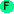car list First element of `list`. ```>(car '(1 2 3)) 1 ```cdr list Remainder of `list`. ```>(cdr '(1 2 3)) (2 3) ```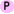caar list Equivalent to `(car (car list))`. ```>(caar '((1 2) 3 4)) 1 ```cadr list Equivalent to `(car (cdr list))`. ```>(cadr '((1 2) 3 4)) 3 ```cddr list Equivalent to `(cdr (cdr list))`. Note that `cdar` is not part of Arc. ```>(cddr '((1 2) 3 4)) (4) ```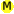conswhen f x y Cons `x` and `y` if `(f x)` is true. Otherwise returns `y`. ```>(conswhen (fn (_) (< (len _) 3)) '(1 2) '(3 4 5)) ((1 2) 3 4 5) ``` ```>(conswhen (fn (_) (< (len _) 3)) '(1 2 3 4) '(3 4 5)) (3 4 5) ```consif x list Cons `x` onto `list` if `x` is not `nil`. ```>(consif 1 '(2 3)) (1 2 3) ``` ```>(consif nil '(2 3)) (2 3) ```firstn n list Returns the first `n` elements of `list`. ```>(firstn 3 '(1 2)) (1 2) ``` ```>(firstn 3 '(a b c d e)) (a b c) ```nthcdr n list Returns `list` after skipping the first `n` elements. ```>(nthcdr 0 '(1 2 3)) (1 2 3) ``` ```>(nthcdr 2 '(1 2 3)) (3) ``` ```>(nthcdr 10 '(1 2 3)) nil ```last list Returns the last element of `list`. ```>(last '(1 2 3)) 3 ```flat list Flattens `list` into a list of atoms. Any `nil`s are removed. ```>(flat '(1 2 () (3 4 (5)))) (1 2 3 4 5) ```rev list Reverses `list`. ```>(rev '(1 2 3)) (3 2 1) ``` ```>(rev '(1 (2 3) 4)) (4 (2 3) 1) ```carif x Returns `(car x)` if `x` is a list, and returns `x` otherwise. This provides a 'safe' way to return the first element of something that may or may not be a list. ```>(carif '(1 2)) 1 ``` ```>(carif 3) 3 ```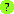caris x val Tests if `x` is a list and `(car x)` is `val`. ```>(caris '(1 2) 1) t ``` ```>(caris 1 1) nil ```intersperse x list Inserts `x` between elements of `list`. If `list` has fewer than 2 elements, there is no effect. ```>(intersperse 1 '(a b (c d) e)) (a 1 b 1 (c d) 1 e) ``` ```>(intersperse nil '(1 2 3)) (1 nil 2 nil 3) ```split list pos Splits `list` into two lists at the given position, which must be no more than the length of the list. ```>(split '(a b c) -1) ((a b) (a b c)) ``` ```>(split '(a b c) 0) (nil (a b c)) ``` ```>(split '(a b c) 1) ((a) (b c)) ``` ```>(split '(a b c) 2) ((a b) (c)) ``` ```>(split '(a b c) 3) ((a b c) nil) ```pair list [f] Splits `list` into pairs. By default, each pair is made into a list. If specified, function f is applied to each pair. ```>(pair '(a b c d)) ((a b) (c d)) ``` ```>(pair '(a b c d e)) ((a b) (c d) (e)) ``` ```>(pair '(1 2 3 4) +) (3 7) ``` ```>(pair '(10 2 3 40 50 6) max) (10 40 50) ```tuples list [n] Splits `list` into groups of `n`. `tuples` is a generalization of `pair`. ```>(tuples '(1 2 3 4 5) 1) ((1) (2) (3) (4) (5)) ``` ```>(tuples '(1 2 3 4 5)) ((1 2) (3 4) (5)) ``` ```>(tuples '(1 2 3 4 5) 3) ((1 2 3) (4 5)) ```join [list ...] Joins lists into one list. ```>(join '(1 2) nil '(3 4)) (1 2 3 4) ```list [arg ...] Creates a list of the arguments. ```>(list 1 2 3) (1 2 3) ``` ```>(list "a" '(1 2) 3) ("a" (1 2) 3) ```copylist args Identical to list. ```>(copylist '(1 2 3)) (1 2 3) ``` ```>(copylist '("a" (1 2) 3)) ("a" (1 2) 3) ```range start end Creates a list of numbers from start to end in steps of 1. The last number is <= end. ```>(range 0 10) (0 1 2 3 4 5 6 7 8 9 10) ``` ```>(range 1.5 3.8) (1.5 3) ```n-of n expr Evaluates `expr` `n` times and returns a list of the results. ```>(n-of 5 "a") ("a" "a" "a" "a" "a") ``` ```>(w/instring ins "abcdefg" (n-of 5 (readc ins))) (#\a #\b #\c #\d #\e) ```adjoin elt list [test] Cons `elt` onto `list` unless `(test elt y)` is true for some `y` in `list`. By default, `test` is `iso`, so `elt` will be joined if it is not present in `list`. ```>(adjoin 2 '(1 2 3)) (1 2 3) ``` ```>(adjoin 2 '(1 3 5)) (2 1 3 5) ``` ```>(adjoin 2 '(1 2 3) <) (1 2 3) ``` ```>(adjoin 2 '(0 1 2) <) (2 0 1 2) ```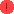counts list [table] Counts how many times each element of `list` occurs. The results are returned as a table mapping from the element to the count. If a table is passed in, it will be updated. ```>(counts '(b a n a n a)) #hash((a . 3) (b . 1) (n . 2)) ``` ```>(let tl (table) (counts '(1 2) tl) (counts '(1 3) tl)) #hash((1 . 2) (2 . 1) (3 . 1)) ```commonest list Returns the element of `list` occurring most frequently, along with its count. ```>(commonest '(b a n a n a)) (a 3) ``` ```>(commonest nil) (nil 0) ```

## Applying functions to lists

Arc provides several ways of applying functions to the elements of a list.reduce f list Reduces `list` using `f`. Applies `f` to the first two elements of `list`. Then recursively applies `f` to that result and the next element of `list`. ```>(reduce + '(1 2 3 4 5)) 15 ``` ```>(reduce + '("a" "b" "c")) "abc" ``` ```>(reduce / '(1 2 3)) 1/6 ```rreduce f list Reduces `list` using `f` in reverse. Applies `f` to the last two elements of `list`. Then recursively applies `f` to that result and the previous element of `list`. ```>(rreduce + '(1 2 3 4 5)) 15 ``` ```>(rreduce / '(1 2 3)) 3/2 ```retrieve n f list Returns the first `n` elements of `list` for which predicate `f` is true. Renamed from firstn-that in arc3. ```>(retrieve 3 odd '(1 2 3 4 5 6 7 8)) (1 3 5) ``` ```>(retrieve 3 odd '(2 4 6 8)) nil ```most f list Returns the element of `list` for which rating function `f` returns the largest value. ```>(most len '("cat" "bird" "dog")) "bird" ``` ```>(most abs '(3 -10 5)) -10 ``` ```>(most abs '(-1 1 -1)) -1 ```map1 f list Applies `f` to the elements of `list`. The results are cons'd together into a list. ```>(map1 (fn (_) (list _ (* _ 10))) '(1 2 3)) ((1 10) (2 20) (3 30)) ``` ```>(map1 cdr '((1) (2 3) (4 5))) (nil (3) (5)) ```mappend f [list ...] Maps `f` on the arguments, and then joins the results together. `f` must return a list. `nil` results are omitted. ```>(mappend (fn (_) (list _ (* _ 10))) '(1 2 3)) (1 10 2 20 3 30) ``` ```>(mappend cdr '((1) (2 3) (4 5))) (3 5) ```reclist f list Recursively applies f to tail subsequences of `list` and returns the first true result. Returns `nil` if none. ```>(reclist (fn (x) (prn x) nil) '(a b c)) (a b c) (b c) (c) nil ``` ```>(reclist (fn (_) (if (is (len _) 2) _)) '(a b c)) (b c) ```mem test list Tests elements of list. If test is true for an element, returns the remainder of the list from that point. `test` is either an element or a predicate. ```>(mem (fn (_) (odd _)) '(2 4 5 6 7)) (5 6 7) ``` ```>(mem 6 '(2 4 5 6 7)) (6 7) ```trues f list Maps function `f` onto `list` and returns only the true (non-nil) values. ```>(trues cdr '((1 2) (3) (4 5))) ((2) (5)) ``` ```>(trues (fn (_) (if (odd _) (* 10 _))) '(1 2 3 4 5)) (10 30 50) ```

## Sorting

Arc provides an efficient sorting operation based on merge sort. Sorting in Arc uses a `compare` predicate function that defines the sort order. Elements `x` and `y` are defined as sorted if `(compare x y)` is true. The `compare` function does not need to define a full order. That is, it is valid for `(compare x y)` and `(compare y x)` to both be true. In this case, `mergesort` is stable, and will preserve the existing order of the elements.mergesort compare list Destructively sorts `list` using the given comparison function. The sort is stable; if two elements compare as equal with `compare`, they will remain in the same order in the output. The original list is destroyed. ```>(mergesort < '(3 0 10 -7)) (-7 0 3 10) ``` ```>(mergesort (fn (a b) (< (len a) (len b))) '("horse" "dog" "elephant" "cat")) ("dog" "cat" "horse" "elephant") ```merge compare list1 list2 Merges two sorted lists into a sorted list. The original lists must be ordered according to the predicate function `compare.` ```>(merge < '(1 2 3 5) '(2 4 6)) (1 2 2 3 4 5 6) ``` ```>(merge (fn (a b) (> (len a) (len b))) '("aaa" "b") '("cccc" "ddd" "ee")) ("cccc" "aaa" "ddd" "ee" "b") ```insert-sorted compare elt list Creates a new list with `elt` inserted into the sorted list `list`. The original list must be sorted according to the comparison function. The original list is unmodified. ```>(insert-sorted > 5 '(10 3 1)) (10 5 3 1) ``` ```>(insert-sorted > 5 '(10 5 1)) (10 5 5 1) ```insort (compare elt list) Insert `elt` into previously-sorted `list`, updating `list`. ```>(let x '(2 4 6) (insort < 3 x) x) (2 3 4 6) ```reinsert-sorted compare elt list Creates a new list with `elt` inserted into the sorted list `list` if it is not already present. The original list must be sorted according to the comparison function. The original list is unmodified. ```>(reinsert-sorted > 5 '(10 3 1)) (10 5 3 1) ``` ```>(reinsert-sorted > 5 '(10 5 1)) (10 5 1) ```insortnew (compare elt list) Insert `elt` into previously-sorted `list` if it is not present, updating `list`. ```>(let x '(2 4 6) (insortnew < 3 x) x) (2 3 4 6) ```best compare list Returns the 'best' element of `list` as determined by function `compare`. ```>(best > '(3 1 4 5 9 6)) 9 ```bestn n compare list Returns the first `n` elements of `list` when sorted according to comparison function `compare`. ```>(bestn 3 > '(3 1 4 5 9 6)) (9 6 5) ``` ```>(bestn 3 < '(3 1 4 5 9 6)) (1 3 4) ```sort test seq Sorts the sequence (list or string) using the specified comparison function. The original sequence is unmodified. ```>(sort < '(3 1 4 9 0)) (0 1 3 4 9) ``` ```>(sort > "Test word") "wtsroedT " ```

## Sequence manipulation

These operations act on lists, strings, or hash tables.sref seq value index Sets indexed entry in a list, string, or hash table to the given value. ```>(let x (copy "abc") ; make the string literal mutable (sref x #\d 1) x) "adc" ``` ```>(do (= x '(1 2 3)) (sref x 4 1) x) (1 4 3) ```count test seq Counts the number of elements of `seq` that satisfy `test`. `test` is an object or predicate. For a table, the elements are the values. ```>(count #\a "banana") 3 ``` ```>(count (fn (_) (odd _)) '(1 2 3 4 5)) 3 ```union f xs ys Takes union of sequence `xs` and `ys`. Predicate `f` is used to determine equality to filter out duplicates. `xs` and `ys` must both be lists or strings. ```>(union is '(1 2 3) '(2 3 4)) (1 2 3 4) ``` ```>(union is "ab" "banana") "abnn" ``` ```>(union (fn (a b) (is (mod a 10) (mod b 10))) '(1 2 3) '(13 24 35)) (1 2 3 24 35) ```len seq Computes the length of `seq`. ```>(len "abc") 3 ``` ```>(len '(1 2 3)) 3 ``` ```>(len (obj a 1 b 2)) 2 ```len< seq n Tests if length of `seq` is less than `n`. ```>(len< "abc" 4) t ``` ```>(len< '(1 2 3) 4) t ``` ```>(len< (obj a 1 b 2) 4) t ```len> seq n Tests if length of `seq` is greater than `n`. ```>(len> "abc" 4) nil ``` ```>(len> '(1 2 3) 4) nil ``` ```>(len> (obj a 1 b 2) 4) nil ```dedup seq Returns contents of `seq` without duplicates. For a string, returns a list of characters. For a table, returns a list of values. ```>(dedup '(1 2 3 2 1)) (1 2 3) ``` ```>(dedup "abcba") (#\a #\b #\c) ``` ```>(dedup (obj a 1 b 2 c 1)) ((a 1) (b 2) (c 1)) ```single list Returns true if given a list of length one. ```>(single '(1)) t ``` ```>(single 1) nil ``` ```>(single '()) nil ```pos test seq [start] Returns the index of the first element of `seq` that satisfies `test`. `seq` is a list or string. `test` is either an object or predicate function. If `start` is given, testing starts at that element. ```>(pos 'c '(a b c d)) 2 ``` ```>(pos #\c "abcd") 2 ``` ```>(pos #\c "abcdc" 3) 4 ``` ```>(pos odd '(2 4 5 6 7)) 2 ``` ```>(pos odd '(2 4 6)) nil ```before t1 t2 seq [start] Tests if `t1` is true before `t2` in `seq`. `seq` is either a list or string. The tests are either objects or predicate functions. If `start` is given, search starts with the specified element. ```>(before 4 odd '(2 4 1 3)) t ``` ```>(before 4 odd '(2 3 4 1)) nil ``` ```>(before #\a #\n "banana") t ``` ```>(before #\a #\n "banana" 2) nil ```rand-elt seq Returns a random element from a list, or a random character from a string. It also works on a table with integer keys from 0 to n. Renamed from random-elt in arc3. ```>(rand-elt '(1 2 3)) 2 ``` ```>(rand-elt "abcd") #\d ```mismatch s1 s2 Compares sequences and returns the position of the first mismatch (as determined by `is`). Returns `nil` if the sequences are identical. ```>(mismatch "abcde" "abXde") 2 ``` ```>(mismatch '(1 2 3) '(1 9 3)) 1 ``` ```>(mismatch "abc" "abc") nil ```find test seq Finds and returns the first element of `seq` that satisfies `test` (object or predicate). `seq` can be a list or string. ```>(find odd '(2 3 4 5)) 3 ``` ```>(find odd '(2 4 6)) nil ``` ```>(find alphadig "...abc...") #\a ```cut seq start [end] Returns subsequence of `seq` from `start` to `end`. If `end` is not specified, the remainder of `seq` is used. The seq can be a list or string. ```>(cut "abcde" 2) "cde" ``` ```>(cut "abcde" 2 4) "cd" ``` ```>(cut '(a b c d) 2) (c d) ``` ```>(cut '(a b c d) 2 4) (c d) ```rem test seq Removes elements from `seq` that satisfy `test`. `test` is either a function or an object. `seq` is either a list or string. ```>(rem odd '(1 2 3 4 5)) (2 4) ``` ```>(rem 3 '(1 2 3 4 5)) (1 2 4 5) ``` ```>(rem #\c "abcde") "abde" ``` ```>(rem (fn (_) (in _ #\a #\b)) "abcde") "cde" ```keep test seq Keeps elements from `seq` that satisfy `test`. `test` is either a function or an object. `seq` is either a list or string. ```>(keep odd '(1 2 3 4 5)) (1 3 5) ``` ```>(keep 3 '(1 2 3 4 5)) (3) ``` ```>(keep #\c "abcde") "c" ``` ```>(keep (fn (_) (in _ #\a #\b)) "abcde") "ab" ```map f [seq ...] Applies `f` to the elements of the sequences, taking the first from each, the second from each, and so on. If there are `n` sequences, `f` must be a function accepting `n` arguments. The sequences can be lists or strings. If any sequence is a string, then `f` must return a character, and the result will be a string made up of the results from `f`. Otherwise, the result will be a list of the results from `f`. The sequences are processed up to the length of the shortest sequence. For a single list, `map` is the same as `map1`. ```>(map (fn (a b c) (+ (* a 100) (* b 10) c)) '(1 2 3) '(4 5 6) '(7 8 9 10)) (147 258 369) ``` ```>(map (fn (_) (list _ (* _ 10))) '(1 2 3)) ((1 10) (2 20) (3 30)) ``` ```>(map cdr '((1) (2 3) (4 5))) (nil (3) (5)) ``` ```>(map (fn (c n) (coerce (+ n (coerce c 'int)) 'char)) "abc" '(0 2 4)) "adg" ``` ```>(map min "bird" "elephant") "bied" ```sum f seq Applies f to the elements of the sequence and sums the results. New in arc3. ```>(sum int "abc") 294 ``` ```>(sum log '(1 2 3)) 1.791759469228055 ``` ```>(sum cadr (obj a 1 b 2 c 3)) 6 ```get index Generates a function to get the element referenced by index; the function can be applied to a table. This is useful for mapping, for instance. (It can also be applied to functions, not jus sequences.) New in arc3. ```>(map get.2 '((a b c) (1 2 3) (p q r))) (c 3 r) ``` ```>(get!b (obj a 10 b 20)) 20 ``` ```>(get.42 log) 3.7376696182833684 ```

## Otherrand-choice expr [...] Randomly choose one of the expressions. ```>(rand-choice "a" 42 '(1 2 3)) (1 2 3) ```compare comparer scorer Creates a procedure on two values that applies `scorer` to each value, and then applies `comparer` to the two scores. ```>(compare < len) # ``` ```>((compare < len) "yz" "abc") t ```only f Creates a procedure that will apply `f` to its arguments only if there are arguments. ```>(only +) # ``` ```>((only +) 1 2 3) 6 ``` ```>((only +)) nil ```accum accfn [body ...] Executes body. Inside body, each time accfn is called, its argument is pushed on a list that becomes the return value. ```>(accum accfn (each x '(1 2 3) (accfn (* x 10)))) (10 20 30) ```summing sumfn [body ...] Sums the number of times sumfn is called with a true argument in body. The sum is returned. The sumfn argument specifies the name under which the summing function is available to the body. ```>(summing istrue (map istrue '(1 nil nil t))) 2 ```